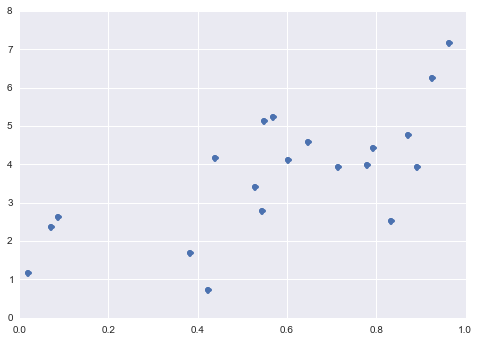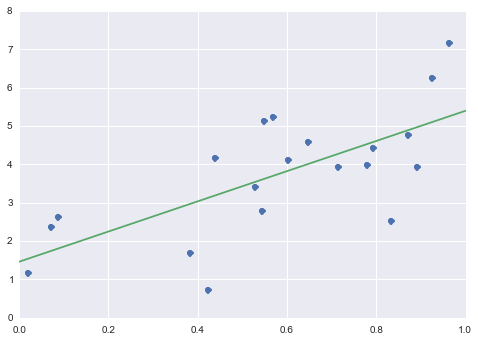# scikit-learn-linear-reg¶

Credits: Forked from PyCon 2015 Scikit-learn Tutorial by Jake VanderPlas

• Linear Regression
In :
%matplotlib inline
import numpy as np
import matplotlib.pyplot as plt
import seaborn;
from sklearn.linear_model import LinearRegression
import pylab as pl

seaborn.set()


## Linear Regression¶

Linear Regression is a supervised learning algorithm that models the relationship between a scalar dependent variable y and one or more explanatory variables (or independent variable) denoted X.

Generate some data:

In :
# Create some simple data
import numpy as np
np.random.seed(0)
X = np.random.random(size=(20, 1))
y = 3 * X.squeeze() + 2 + np.random.randn(20)

plt.plot(X.squeeze(), y, 'o');Fit the model:

In :
model = LinearRegression()
model.fit(X, y)

# Plot the data and the model prediction
X_fit = np.linspace(0, 1, 100)[:, np.newaxis]
y_fit = model.predict(X_fit)

plt.plot(X.squeeze(), y, 'o')
plt.plot(X_fit.squeeze(), y_fit);